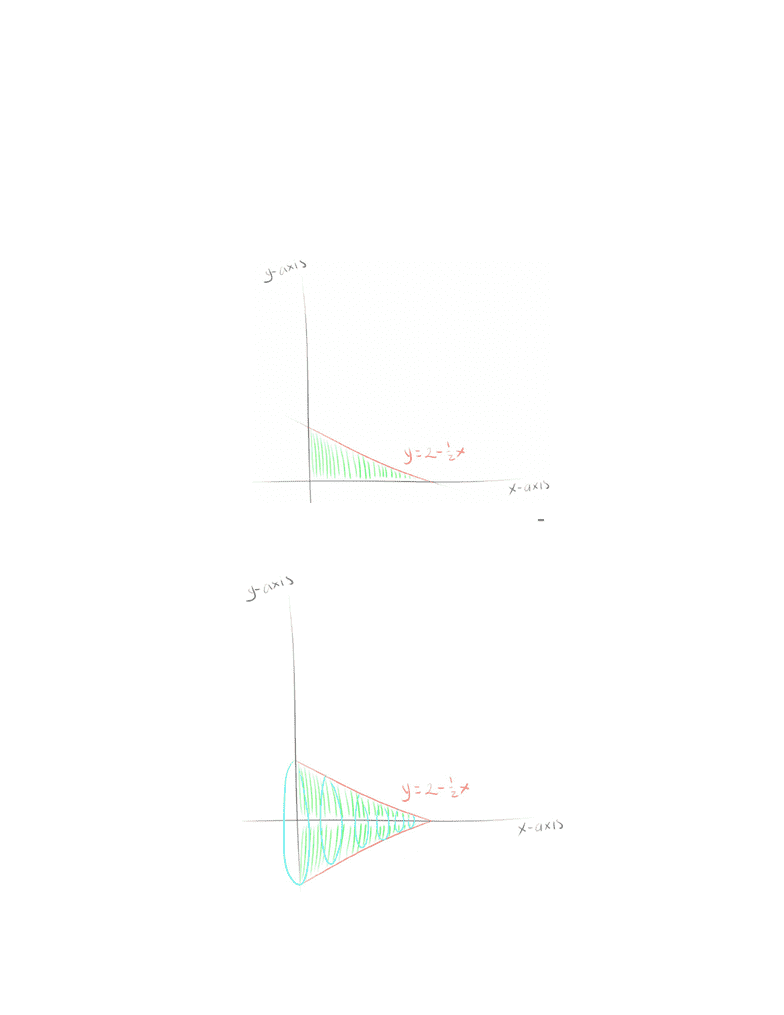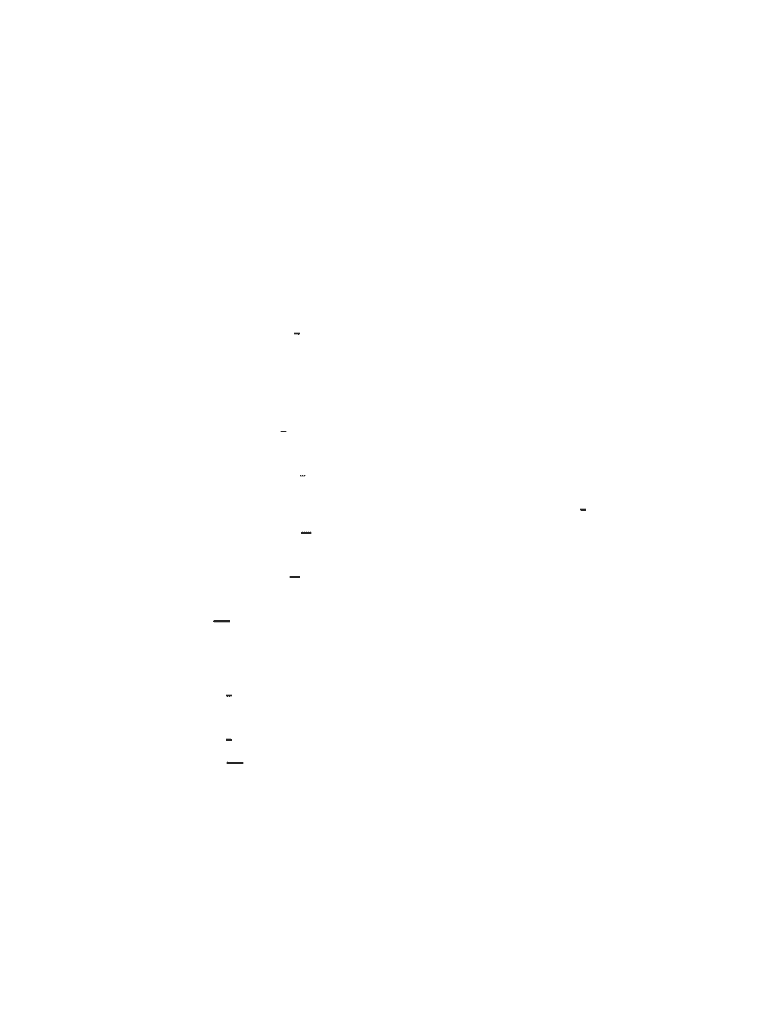Study Guides (390,000)
US (220,000)
UC-Irvine (3,000)
MATH (600)
MATH 2B (100)
All (60)
Midterm

# MATH 2B Midterm: MATH2B, Chapter 6.1 term test 1Premium

Department
Mathematics
Course Code
MATH 2B
Professor
All
Study Guide
Midterm

This preview shows pages 1-2. to view the full 7 pages of the document.Volume
How would you take the 3D volume of a 2D function?
Rotate it around a line
Example
How would you take the volume of the area of 2 x,x xis and y xisy = 2
1 aa
around the x-axis?
What would it look like?
The volume created by the area rotated around the x-axis looks like a cone
How can we find the volume?
Volumes
Section 6.2

Only pages 1-2 are available for preview. Some parts have been intentionally blurred.

Subscribers OnlyYou can use integrals to find the volume of the cone
What is the area of a circle?
rπ2
What would the boundaries be?
The x and y intercepts are where the function intercepts with the other
lines
So 0, x 4x= =
The function itself is the radius
(2 x)r= 2
1
πrΔxV = 2
(2 ) dxV =
4
0
π 2
12
π 2x x dx=
4
0
4 + 4
1 2
Here, you can move outside the integral and foil out π 2 )( 2
12
π(4x x x) (between 0 and 4)= 2+ 1
12 3
Then take the integral
π(16 6 ) π(0 0 0)= 1 + 12
64 +
Plugging in the boundaries
=3
16π
Now you can check whether this is true using the actual formula for Volume of a cone
πr HV = 3
12
Plug in the necessary components
)(2) (4)V= 3
12
V=3
16π